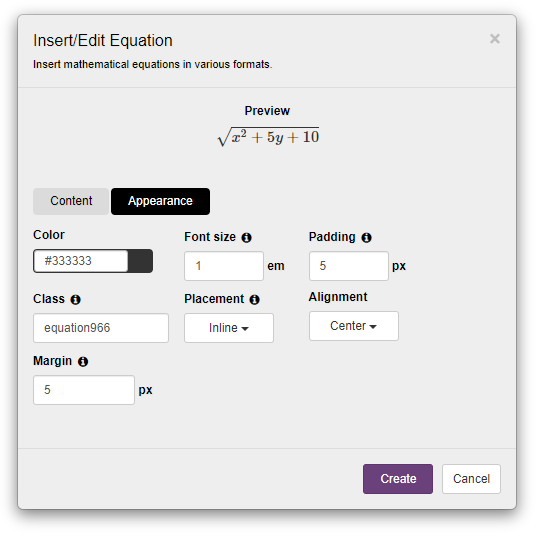Learn how to add mathematical equations in different formats.

Add math equations easily using the Equation tool. You can insert math equations using either AsciiMath, MathML, or LaTeX. Each of these 3 writing styles (or markup languages) gives you high precision and control over creating complex math equations.